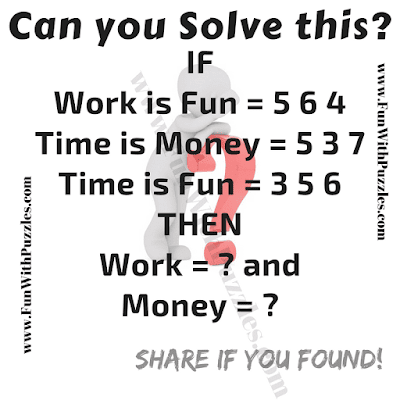## Friday, August 17, 2018

This is very Interesting Maths Picture Puzzle. In this Fun Maths Picture Puzzle, there are some English sentences which equates to some digits. Your challenge here is to decode the value of the each given word. Lets see how much time you will take to solve this Fun Maths Picture Puzzle?Can you solve this Fun Maths Picture Puzzle?

Answer of this "Fun Maths Picture Puzzle", can be viewed by clicking on button. Please do give your best try before looking at the answer.

1.2+1=9
4+2=18
2+9=9
6+2=32
3+3=12
Then
6+4=??

2.21=4
13=6
23=12
34=24
Then what is
25=?

1.Answer to this puzzle is 20.
If AB=C is the given number equation, then the logical reasoning is as below
C=AxBx2

A comment doesn't cost a thing. Please drop a comment below to boost the author's morale.